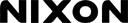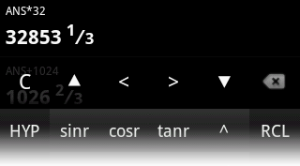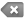# EvalInfix Calculator

Help and Information > Infix Calculator

## Introduction

Infix Calculator works like a physical scientific calculator. Expressions are entered like they would be written.

### Examples

• sin(pi/3+2pi*32) = 0.8660254037844377
• deriv(X^2,X,.01) = 9.999999999999787
• ln(-1) = 3.1415926535898i [Complex]

### Modes of Operation

To change the mode, enable/disable Complex Numbers in Preferences.

• Normal - Calculations involving real numbers only. All functions are enabled.
• Complex - Calculations involving both complex numbers and real numbers. Only functions accepting complex arguments are enabled.

When an expression is evaluated successfully, its result is stored in variable ans (Last Answer). Pressing the [+],[−],[×], or [÷] keys when the cursor is at the beginning of the expression will insert ans at the beginning of the expression. To prevent this from happening for negation, press [INV] then [(-)].

## Interface

### Editing ButtonsC, CA Clear/Clear All [CA] Clears last answer as well as all user-defined variables. ▲ ▼ Previous/Next Expression <  > Move Cursor Left/RightDelete

### Evaluation History

The topmost area is the list of all previous evaluations. This list can be scrolled. Scroll down to hide all buttons. To show the buttons again, scroll up, click the input box, or press [BACK] on your device.

• To insert an expression from the list into the input box, long press the expression and choose [Insert Expression] from the resulting menu.
• To store the result of an expression from the list, long press the expression and choose [Store Result] from the resulting menu.

### Display Mode

Click on any evaluation to change the display mode. Possible display modes are as follows:

Real Numbers
Normal Mode Displays numbers as decimals.
Fraction Mode Displays numbers as improper fractions when possible. If fractions are approximated, they will be displayed mixed.
Mixed Fraction Mode Displays numbers as mixed fractions when possible.
Complex Numbers
Complex Form a+bi Displays complex numbers in the form a+bi.
Fraction Mode Displays complex numbers in the form a+bi as fractions, e.g.: 2/3+4/5i
Complex Form re^Θi Displays complex numbers in polar form, e.g.: 1.0 e0.7853981633974482i

## Notes

### General Notes

• The Minus key [−] is used for both subtraction and negation.
• Multiplication operator (*) can be omitted: before constants, before + after functions and parentheses.
2pisin(x)cos(x) = 2*pi*sin(x)*cos(x)
• Parentheses do not need to be matched.

1-2)(3+4 = (1-2)*(3+4)
(1-2(3+4 = 1-2*(3+4)

### Internal Fractions

Numbers are stored internally as fractions when possible. There is no separate fraction button to enter fractions. Use the Divide key [÷] to enter fractions. Numbers up to 6 decimal places in length are also converted into fractions.

## Variables and Constants

A, B, C, X, Y, Z

#### User-defined Variables

Can be modified by pressing [STO] followed by variable in calculator, or by using the sto() function. Can be fractions, complex numbers, and real numbers.
x, y, z, t, v, r, θ m

#### Volatile Variables

Modified by graphing and table features such as tracing.
Variables x, y are always modified. Variable m (Derivative) is calculated for 2D functions.

• In 3D function mode, z is also modified.
• In 2D parametric mode, t is also modified.
• In 3D parametric mode, z, t, and v are also modified.
• In 2D polar mode, r and θ are also modified.
Cannot be fractions or complex numbers.
ANS

In Infix Calculator, result of previous calculation is stored in this variable. Can be fraction, complex number, or real number.

c, h, G

#### Physical Constants

Speed of Light, Planck's Constant, Gravitational Constant
F, L, R

i, π or pi, phi

#### Mathematical Constants

Imaginary Unit, pi, Golden Ratio
• To recall a user-defined variable, press the Recall [RCL] key, followed by variable in list that appears.
• To store a number into a user-defined variable, press the Store [STO] key, followed by variable in list that appears.
• Complex numbers may be stored, but they cannot be used in normal mode.

## Preferences

Angle Unit Adjusts measurement of angles. Applies to infix calculator, RPN calculator, graphs, and tables.
• Degrees
Applies to standard trigonometric functions sine, cosine, tangent, arcsine, arccosine, arctangent.
Current angle unit is indicated by last letter on button for each function. E.g.: sinr, cosr, tanr for radians.
Complex Numbers Enable Complex Numbers. To adjust form of displayed complex numbers...

#### Fraction Approximations

Estimate fractions when interal fractions cannot be used.
Vibrate Vibrate when a key is pressed.
Live Calculation Calculate as an expression is entered. To register an expression, press the Equals [=] button. (Does not apply to RPN mode.)

## Errors

Math Errors
Undefined An attempt was made to divide by zero.

#### Examples:

• 0/0, 0^-1
Domain The input of the function is outside the domain of the function.
• binpdf(2,2,1), ln(-2)
Overflow Evaluation is outside maximum allowable range.
• 171!
Nonreal Result In normal mode, a complex number occurred.
• sqrt(-2), i
Singularity Function cannot be evaluated at the given input value.